## Ian's discovery to get any number in Pascal's Triangle

Chapter 9: The Binomial Expansion and Infinite Series
We'll look at the coefficients found in the expansion of (A+B)n above: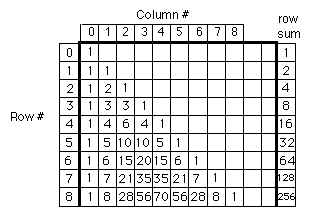For about 35 years my students and I looked at these numbers and found many patterns. For example if you add two numbers in a row, like 4+6, you get the number below the 6 in the in the next row, 10. Looking down column #2, 1,3 6 10 ... They go up 2, then 3 (to get 6), then 4 (to get 10), and so on. So you can predict the number below 10 in the next row. And you can find a rule for each column. What we'd like to do is to be able to find the 8th number in the 20th row, though. What Ian did was something special, and enabled one to do this. Ian did this at age 12. Newton did a similar thing when he was 19, according to W.W. Sawyer in "Integrated Mathematics Scheme- Book C". I just happened to receive this book from England about a week after Ian did his thing. In Ian's words:

"I was faced with the problem of generating Pascal's triangle. I decided to start looking at patterns until I found one that applied to the entire triangle. After some trial and error, I noticed a pattern in the ratios from one column to the next. In row 4, for example, the ratios are arrived at by asking,
what times 1 = 4?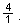What times 4 = 6?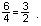Then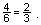And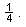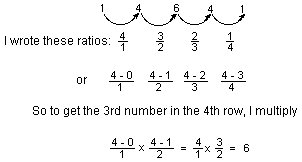To get the 8th number in the 20th row: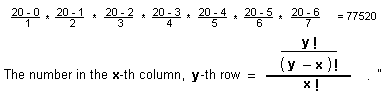Ian switched from the 'number in the row' to 'the column number'. Since the columns start with the 0th column, his x is one less than the number in the row, for example, the 3rd number is in column #2. In other words just subtract 1 first, from the number in the row and use that as x.
Notice that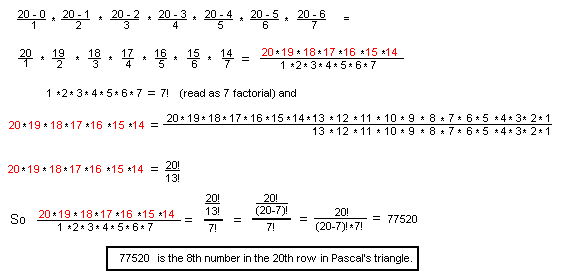And the general term, the number in the xth column, in the nth row, of the binomial expansion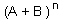is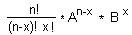We go on to show how this is used to find (1 - x)-1 and later in chapter 10 to find the square root of 2, and later still in ch. 11 to find compound interest and e.
Back to Ch. 9 beginnings
To order Don's materials
To choose sample problems from other chapters
Mathman Home